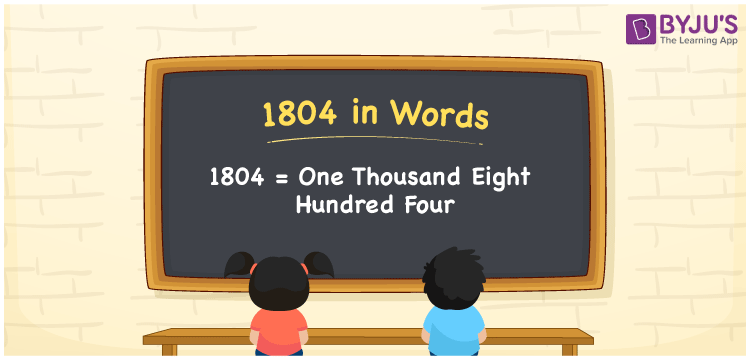# 1804 in Words

We can write 1804 in words as One thousand eight hundred four. If you bought five bunches of bananas for Rs. 1804, then you can say, “I bought five bunches of bananas for One thousand eight hundred four rupees”. Here, One thousand eight hundred four is the number name or word form of the cardinal number 1804. In this article, you will learn how to write the number 1804 in words using a place value chart.

 1804 in words One thousand eight hundred four One thousand eight hundred four in Numbers 1804

## 1804 in English Words

Generally, we express numbers in words using the English alphabet. So, we spell 1804 in words as “One thousand eight hundred four”.## How to Write 1804 in Words?

As we can observe that 1804 contains four digits, let’s create a place value chart in such a way that place value is defined for all the digits of 1804. This can be done as given below.

 Thousands Hundreds Tens Ones 1 8 0 4

Here, ones = 4, tens = 0, hundreds = 8, and thousands = 1.

By expanding these numbers, we get;

1 × Thousand + 8 × Hundred + 0 × Ten + 4 × One

= 1 × 1000 + 8 × 100 + 0 × 10 + 4 × 1

= 1000 + 800 + 4

= One thousand + Eight hundred + Four

= One thousand eight hundred four

Therefore, 1804 in words = One thousand eight hundred four.

1804 is a natural number that precedes 1805 and succeeds 1803.

1804 in words – One thousand eight hundred four

Is 1804 an odd number? – No

Is 1804 an even number? – Yes

Is 1804 a perfect square number? – No

Is 1804 a perfect cube number? – No

Is 1804 a prime number? – No

Is 1804 a composite number? – Yes

## Frequently Asked Questions on 1804 in Words

Q1

### How do you say 1804 in words?

We can spell the number 1804 in English words as “One thousand eight hundred four”.
Q2

### How to write Rs. 1804 in words on a cheque?

On a cheque, Rs. 1804 can be written in words as “One thousand eight hundred four rupees only”.
Q3

### What number is One thousand eight hundred four in words?

One thousand eight hundred four = 1000 + 800 + 4 = 1804 Therefore, 1804 is written as One thousand eight hundred four in words.